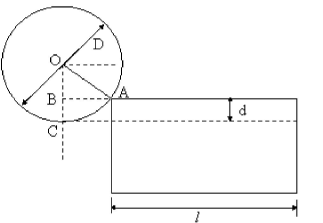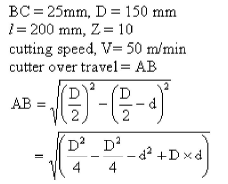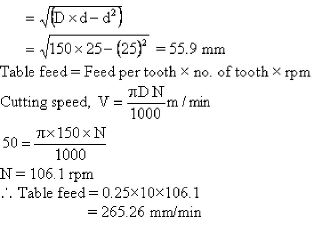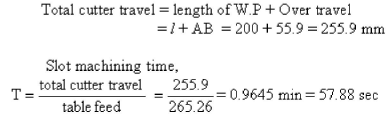Courses

# Production And Engineering Materials - 1

## 10 Questions MCQ Test Mock Test Series - Mechanical Engineering (ME) for GATE | Production And Engineering Materials - 1

Description
This mock test of Production And Engineering Materials - 1 for GATE helps you for every GATE entrance exam. This contains 10 Multiple Choice Questions for GATE Production And Engineering Materials - 1 (mcq) to study with solutions a complete question bank. The solved questions answers in this Production And Engineering Materials - 1 quiz give you a good mix of easy questions and tough questions. GATE students definitely take this Production And Engineering Materials - 1 exercise for a better result in the exam. You can find other Production And Engineering Materials - 1 extra questions, long questions & short questions for GATE on EduRev as well by searching above.
QUESTION: 1

Solution:
QUESTION: 2

Solution:
QUESTION: 3

### The metal powder used in thermit welding of steel is

Solution:
QUESTION: 4

Oxyacetylene reducing flame is used while carrying out the welding on

Solution:

Oxyacetylene reducing flame is used while carrying out the welding on mild steel.

QUESTION: 5

The purpose of chaplets is

Solution:
QUESTION: 6

Match List - I (Name of the Element) with List - II (Crystal Structure) and select the correct answer using the codes given below the lists:

L ist - I
P. Fluorspar
Q. Alpha-Iron
R. Silver
S. Zinc

List - II
1. Body-centered cubic
2. Hexagonal close packed
3. Simple cubic
4. Face-centered cubic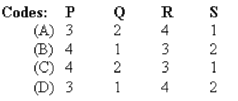Solution:
QUESTION: 7

Welding of C40 steel plate of 10 mm thickness required a current of 150 amps, while it was 350 amps when the plate thickness was increased by 50%. Estimate the welding current for 8.25 mm thickness of same material.

Solution:

Given,
Current required for 10 mm thick plate =150 amps
Current required for 15mm thick plate = 350 amps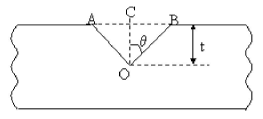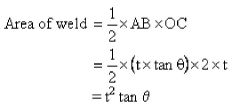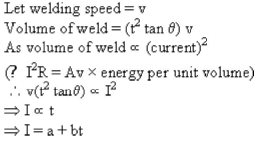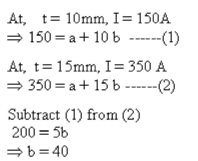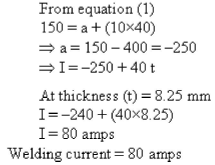QUESTION: 8

In gas welding of mild steel using an oxy-acetylene consumed was 10 liters. The oxygen consumption from the cylinder is

Solution: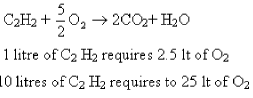*Answer can only contain numeric values
QUESTION: 9

A spot welding obtained with a current of 40000 A for a period of 0.01 sec. If the effective resistance of the joint be taken as 120 micro ohms, and if the joint can be considered as a cylinder of 6mm diameter and 3 mm in height, the heat distributed to the surroundings is ________ J. (Take heat re q u ired for m eltin g steel as 10 J/mm3

Solution:

Given,
Current (I) = 40000 A
Time (t1) = 0.01s
Resistance (R) = 120μΩ = 120x 10-6 Ω
Nugget formed is cylindrical with diameter (dn) = 6mm
Height (hn) = 3 mm
Total heat required for melting steel = 10 J/mm3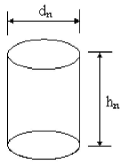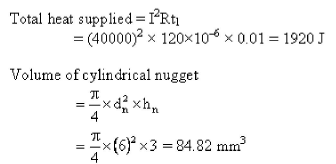So heat required for melting steel = 10x34.82 = 848.2 J
So, heat lost surrounding per nugget = 1920 - 848.2 = 1071.8 J

QUESTION: 10

A slot 25 mm deep is to be cut through a workpiece 200 mm long with the help of HSS side and face cutter whose diameter is 150 mm and has 10 teeth. If cutting speed is 50 m/min and feed is 0.25 mm per teeth, then time (in sec) required to machine the slot is

Solution: